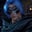Related Tags

golang
communitycreator

# How to check if a value exists in a map in GolangGutha Vamsi Krishna

In this shot, we will learn to check if a value exists in a map using Golang.

No built-in function checks if a value exists in a map in Golang.

So, we will use the following approach to check if a value exists in a map:

• Traverse through the entire map.
• Check if the present value is equal to the value the user wants to check.
• If it is equal and is found, print the value.

• Otherwise, print that the value is not found in the map.

### Example

In this example, we will have a dictionary where the student names are the key, and their ages are the value.

We will check whether or not students of a certain age are present.

### Code

package main

//program execution starts here
func main(){

//declare a map
students := map[string]int{
"john": 10,
"james": 12,
"mary":13,
}

//provide user value
var userValue = 10

//call function and proceed according to return
if checkForValue(userValue,students){
//executes if a value is present in the map
println("Value found")
}else{
//executes if a value is NOT present in the map
}

}

//function to check if a value present in the map
func checkForValue(userValue int, students map[string]int)bool{

//traverse through the map
for _,value:= range students{

//check if present value is equals to userValue
if(value == userValue){

//if same return true
return true
}
}

return false
}



### Explanation

• We declare and initialize a map students, with the key as the student’s name and the value as their age.

• Line 14: We provide user input.

• Line 30: We declare the function that accepts the userValue and students as parameters and returns a boolean value.

• Line 33: We traverse through the map, students, and check if the present value equals the userValue. If it is equal, return true.

• Line 44: If the userValue is not found in the map after traversing, return false.

• Line 17: We call the checkForValue function and use an if statement to check if the returned value is true or false. We then display the output accordingly.

RELATED TAGS

golang
communitycreator

CONTRIBUTORGutha Vamsi Krishna
RELATED COURSES

View all Courses

Keep Exploring

Learn in-demand tech skills in half the time